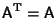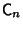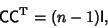## C-Matrix

Any Symmetric Matrix () or Skew Symmetric Matrix ()with diagonal elements 0 and otherssatisfyingwhere I is the Identity Matrix, is known as a-matrix (Ball and Coxeter 1987). Examples includeReferences

Ball, W. W. R. and Coxeter, H. S. M. Mathematical Recreations and Essays, 13th ed. New York: Dover, pp. 308-309, 1987.